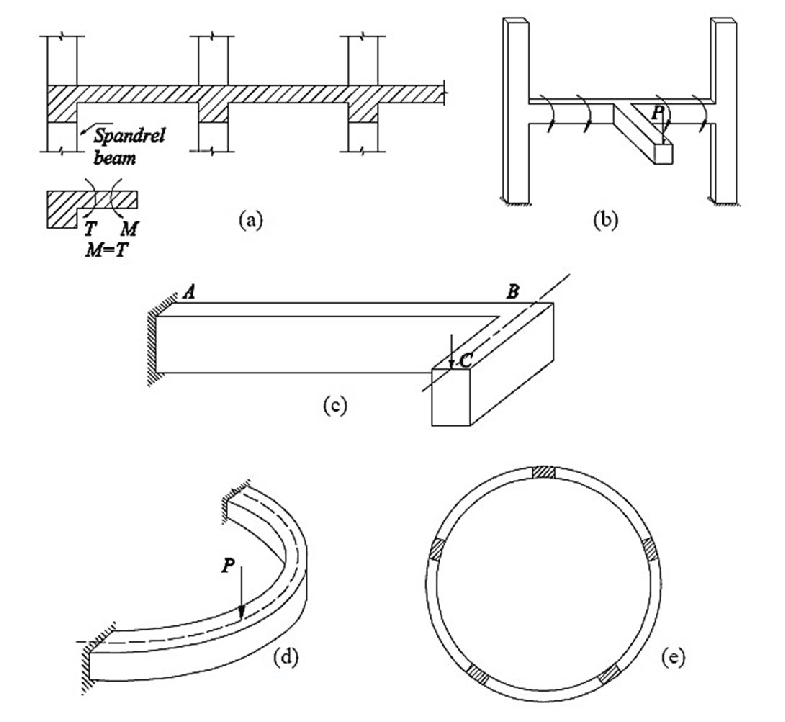### torsional stiffness of members when to considered in analyzing a bridge

Loads acting normal to the bending plane will cause bending moment and shear force when this loads act away from the planes, torsion moment will develop with bending moment and shear force. figure 1 shows different examples of beams subjected to torsion. shear stress due to torsion will cause diagonal cracks. if the member is not reinforced sufficiently, brittle and sudden failure may occur. this forces will act on a member at the same time. analyzing the combined effects of bending moment, shear force and moment is very complex due to the variable of cracks, inelastic behavior of concrete and the complex stress distribution due to the effects of combining bending moment, shear force and torsion. therefore, the member designed separately for torsion. then the required reinforcement is combined with other requirements.Figure 1

Torsional stiffness can be defined as a property of materials determining the rigidity of materials and ability to resist twisting induced by torsion. torsion stiffness can be calculated using the following equation

K=((G*J/L)

where
G is the elastic shear modulus
J is the polar moment of inertia
L is the effective span or length of the member under investigation

For box-girder beam selected due to it is high torsional stiffness and resistance, in this case, torsional resistance should be included in analysis and design.
Due to the complexity accompanied by including torsional stiffness or resistance in design and analysis of the structural member, it is common to ignore torsional resistance, but in this case, higher bending moment will be included in the member
for computer modeling, if the structural member torsional stiffness is added to the model during structural analysis then it is important to check the torsional resistance of the structural members.